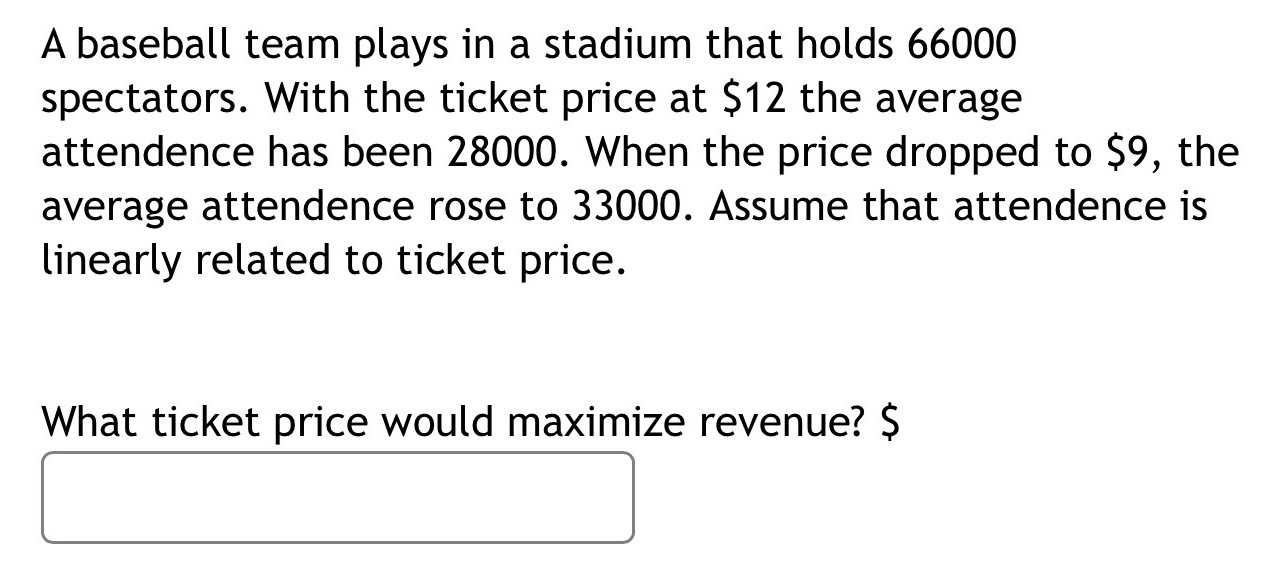### ¿Todavía tienes preguntas de matemáticas?

Pregunte a nuestros tutores expertos
Algebra
PreguntaA baseball team plays in a stadium that holds $$66000$$ spectators. With the ticket price at $$\ 12$$ the average attendance has been $$28000$$ . When the price dropped to $$\ 9 ,$$ the average attendence rose to $$33000$$ . Assume that attendance is linearly related to ticket price.

What ticket price would maximize revenue?